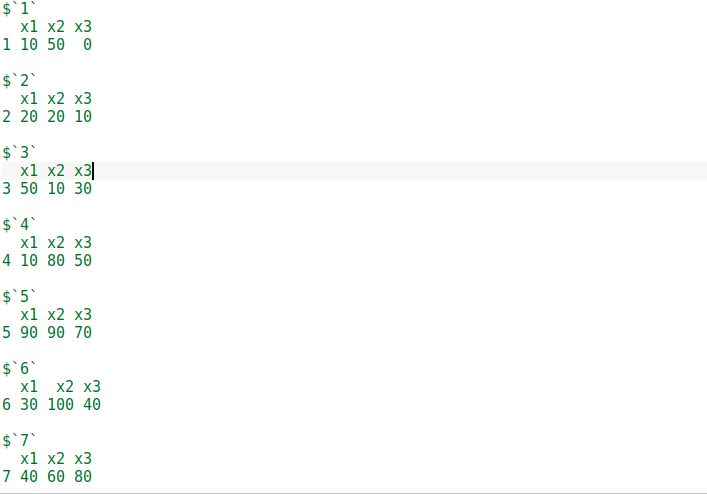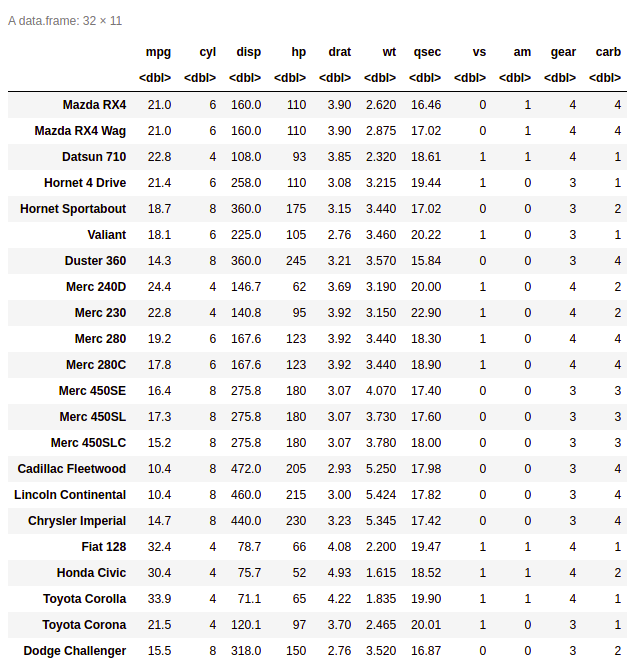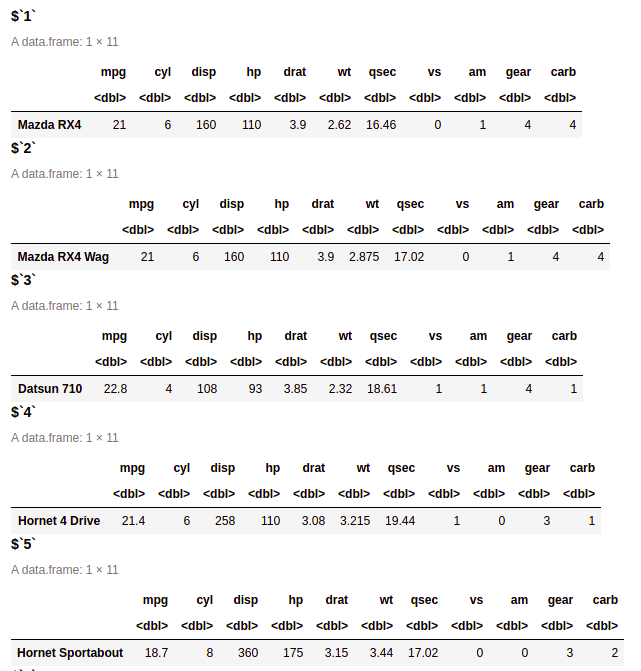# How to convert R dataframe rows to a list ?

In this article, we will see how to convert the rows of DataFrame to the list in R Programming Language.

We can convert a given data frame to a list by row we use the split() function. The split() function is used to split the data according to our requirement and the arguments given in the split() function are data itself and the other one is seq() function. The seq() function is used to generate the sequence of the data and in the seq() function we give one argument i.e. nrow() so, that the sequence is generated row-wise and in nrow() function we give the data as an argument.

Syntax:

split(data, seq(nrow(data)))

Example:

With seq()

## R

 `# Create example data frame ` `data <- ``data.frame``(x1 = ``c``(10,20,50,10,90,30,40),  ` `                  ``x2 = ``c``(50,20,10,80,90,100,60), ` `                  ``x3 = ``c``(0,10,30,50,70,40,80))     ` ` `  `#  print the dataframe ` `data ` ` `  `# Convert rows to list ` `list <- ``split``(data,``seq``(``nrow``(data)))    ` ` `  `# print the list ` `list `

Output:Without using seq():

## R

 `# Create example data frame ` `data <- ``data.frame``(x1 = ``c``(10,20,50,10,90,30,40),  ` `                  ``x2 = ``c``(50,20,10,80,90,100,60), ` `                  ``x3 = ``c``(0,10,30,50,70,40,80))     ` ` `  `#  print the dataframe ` `data ` ` `  `# Convert rows to list ` `list <- ``split``(data,1:``nrow``(data))    ` ` `  `# print the list ` `list  `

Output:Example 2: In this example, mtcars data is used:

## R

 `# Data Frame created ` `data <- ``data.frame``(mtcars)  ` ` `  `# print the data frame ` `data ` ` `  `# Convert rows to list ` `list <- ``split``(data,1:``nrow``(data))   ` ` `  `# print tghe list ` `list `

Output:Whether you're preparing for your first job interview or aiming to upskill in this ever-evolving tech landscape, GeeksforGeeks Courses are your key to success. We provide top-quality content at affordable prices, all geared towards accelerating your growth in a time-bound manner. Join the millions we've already empowered, and we're here to do the same for you. Don't miss out - check it out now!